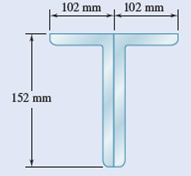Problem

# A compression member of 7-m effective length is made by welding together two L152 × 102 ×...

A compression member of 7-m effective length is made by welding together two L152 × 102 × 12.7 angles as shown. Using E = 200 GPa, determine the allowable centric load for the member if a factor of safety of 2.2 is required.

Fig. P16.13#### Step-by-Step Solution

Solution 1

Obtain the following Properties of the angular section $\mathrm{L} 152 \times 102 \times 12.7$ from the APPENDIX $\mathrm{C}$, "Properties of rolled-steel shapes" from the text book.

Area of the section is, $A=3060 \mathrm{~mm}^{2}$

Moment of inertia of the section about $x$ -axis, $I_{x}=7.20 \times 10^{6} \mathrm{~mm}^{4}$

Moment of inertia of the section about $y$ -axis, $I_{y}=2.59 \times 10^{6} \mathrm{~mm}^{4}$

Centroid of the section is,

$\bar{x}=24.9 \mathrm{~mm}$

$\bar{y}=50.3 \mathrm{~mm}$

Calculate the moment of inertia of the welded section about $x$ axis,

$$I_{x}=2\left(I_{x}\right)_{\text {each }}$$

Substitute $7.20 \times 10^{6} \mathrm{~mm}^{4}$ for $I_{x}$.

\begin{aligned} I_{x} &=2 \times 7.20 \times 10^{6} \\ &=14.4 \times 10^{6} \mathrm{~mm}^{4} \end{aligned}

Calculate the moment of inertia of the welded section about $y$ axis,

$I_{y}=2\left(I_{y}+A x^{2}\right)$

Substitute $2.59 \times 10^{6} \mathrm{~mm}^{4}$ for $I_{y}, 3060 \mathrm{~mm}^{2}$ for $A$, and $24.9 \mathrm{~mm}$ for $x$.

\begin{aligned} I_{y} &=2\left(2.64 \times 10^{6}+3060 \times 24.9^{2}\right) \\ &=8.9744 \times 10^{6} \mathrm{~mm}^{4} \end{aligned}

Hence, the minimum moment of inertia is the minimum of $I_{y}$ and $I_{x}$.

\begin{aligned} I_{\min } &=I_{y} \\ &=8.9744 \times 10^{6} \mathrm{~mm}^{4} \end{aligned}

$$P_{c r}=\frac{\pi^{2} E I_{\min }}{L_{e}^{2}}$$

Here, $E$ is the young's modulus, and $L_{e}$ is the effective length.

Substitute 200 GPa for $E, 8.9744 \times 10^{6} \mathrm{~mm}^{4}$ for $I_{\min }$, and $7 \mathrm{~m}$ for $L_{e}$.

\begin{aligned} P_{\sigma} &=\frac{\pi^{2} \times\left(200 \times 10^{9} \frac{\mathrm{N}}{\mathrm{m}^{2}}\right) \times\left(8.9744 \times 10^{6}\left(10^{-3} \mathrm{~m}\right)^{4}\right)}{\left(7^{2} \mathrm{~m}^{2}\right)} \\ &=361525.62 \mathrm{~N} \\ &=361.525 \mathrm{kN} \end{aligned}

Calculate the allowable using the following relation.

$$P_{\text {allow }}=\frac{P_{\alpha r}}{F . S}$$

Substitute $361.525 \mathrm{kN}$ for $P_{c r}$, and $2.2$ for $F . S$.

\begin{aligned} P_{\text {allow }} &=\frac{361.525}{2.2} \\ &=164.33 \mathrm{kN} \end{aligned}

Therefore, the allowable critical load for the member is $164.33 \mathrm{kN}$.